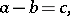# Subtraction

(diff) ← Older revision | Latest revision (diff) | Newer revision → (diff)
The arithmetical operation opposite to addition, i.e. finding one of the terms from the given sum and the given other term. The given sum is named the minuend, the given term is known as the subtrahend, while the term to be found is called the difference. Subtraction is denoted by(minus). Thus, in the expressionis the minuend,is the subtrahend andis the difference.